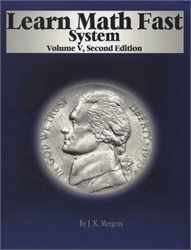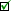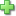# Learn Math Fast System Volume V

## Algebra 1

by J. K. Mergens
Consumable Workbook, 254 pages
Current Retail Price: \$38.00
Used Price: \$24.00 (1 in stock)Condition Policy

Publisher Description:

Included:

• 255-page paperback book with 36 lessons, 36 worksheets, six chapter tests and one final test
• Answer Key in the back of the book with full solutions
• If your student scores well (B+ or better) on the final test, then one credit for Algebra 1 has been earned
• Topics covered in volume 5 are typically taught in tenth grade, but we don't mention grade level in the books

An older student can complete this book in about three months.

I'm looking for an Algebra program.  Can I start with Volume 5?

If your student knows how to find the slope of a line, the y-intercept of a line, and can graph a linear equation, then you may be able to jump into volume 5.  Take the linked .pdf pre-algebra test below to see if your student knows everything taught in the earlier volumes.
If your student is unclear about linear equations, then start with volume 3.  You can skip volume 4, if your student knows the three special triangles and the Pythagorean Theorem, otherwise read volumes 3 - 5 to make it a solid stand-alone algebra curriculum.

Scope and Sequence (Algebra 1):

• Students are taught Algebra Terminology, the difference between Integers, Rational, Irrational, Real and Natural numbers.
• Students will solve equations with Absolute Value.
• Students will solve multiplication and division problems with terms and exponents.
• Algebraic equations will be simplified by combining like term.
• Learn the difference between square roots and cube roots.
• Use the Laws of Algebra to multiply a term by an expression
• Gain knowledge in solving equations with Monomials, Binomials and Trinomials
• Use prime factorization to find cube and square roots.
• Students will Factor out a Common Factor to simplify algebraic equations
• Students will use our quick and easy method to factor a quadratic equation.
• Gain knowledge in a perfect square and how to complete the square in a quadratic equation.
• Learn how to use the Quadratic Formula.
• Get an introduction to Functions.

Did you find this review helpful?
Series Description
Related Categories
Recommended for...
Customer ReviewsClick here to write a review